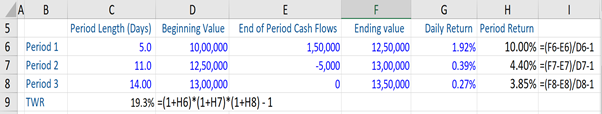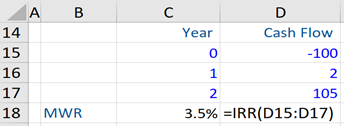## What are “Time Weighted and Dollar Weighted Returns?”

Time Weighted Return (TWR) and Dollar (Money) Weighted Return (MWR) are two methods or approaches to evaluating the performance of a managed investment portfolio over time. The TWR is the compound rate of growth over a stated evaluation period of \$1 initially invested in the account. Whereas the MWR measures the compound growth rate of all funds invested in the account over the evaluation period (it is also known as the “internal rate of return” or IRR). Simply put, the MWR is the discount rate that sets the present value of cash inflows equal to the present value of all cash outflows.

As a result, unlike the TWR, MWR does not only calculate the investment performance, but also takes into account all the cash flows coming in and out of the account. There are particular instances, where each of the two methods is preferred over the other.

## Key Learning Points

• TWR and MWR both measure the performance of a portfolio over time
• Investors prefer a rate of return measure that is not sensitive to cash flows, and this is where the TWR measure is particularly useful
• Under normal conditions, TWR and MWR would produce similar results
• However, if there are large external cash flows the TWR and MWR can look very different

## Time Weighted Return (TWR)

A TWR requires that the account be revalued every time there is an incoming cash inflow or outgoing cash flow. This is done because TWR derives its name from the fact that each sub-period return, the periods between cash flows, receive a weight proportional to the length of the sub-period relative to the full length of the evaluation period. This means that each return is weighted by the time that it was included in that evaluation period.

The formula for TWR is:

TWR = (1+r1) * (1+r2)* (1+rn) – 1

Where r is equal to the return in different periods.

## Time Weighted Return (TWR), Example

For example, assume we have a portfolio over three (3) periods with various beginning and ending values, and various cash flows throughout the period. From here, we first compute the period return (r1, r2, r3) for each of these periods. This calculates the ending value of a period minus any cash flows in that period divided by the beginning value of the period minus 1 as shown below.This eliminates the impact of portfolio cash flows on the returns that are calculated. As a consequence of this, the calculation is not sensitive to the contributions or withdrawals.

In most situations, an investment manager, such as a mutual fund manager, has little or no control over the size or timing of the external cash flows – the investments or redemptions – into and out of the fund. Therefore, in such circumstances, investors generally prefer a rate of return measure which is not sensitive to the cash flows, if they want to evaluate a manager’s actions and how they affected the account value and this is where the TWR comes in.

Now if we link each period’s return and multiply them in a compounding manner, we can calculate the TWR for the portfolio across the three periods – which here is 19.3% (calculated above).

## Money Weighted Return (MWR)

MWR is the discount rate where PV of cash outflows = PV of cash inflows.

The formula for MWR is:

MWR = CF0 + CF1/(1+IRR) + CF2/(1+IRR)^2 + CF3/(1+IRR)^3 +….+ CFn/ (1+IRR)^n

An Excel function can be used for the IRR function, where we would capture all cash flows to calculate the MWR.

Under normal conditions, TWR and MWR would produce similar results. However, when there are large external cash flows relative to the account’s value and the account’s performance fluctuates significantly during the measurement period, then the MWR and TWR can differ substantially.

An instance where MWR measure of account returns is preferable over TWR is when an investment manager does control the timing and the amounts of cash flows into an account. For example, managers of various types of private equity investments or funds have arrangements that allow them to call capital from their investors, and it is usually at the manager’s discretion ultimately when they will return original capital to investors in any earnings on that capital.

## Money Weighted Return (MWR), Example

A stock was purchased for \$100 and sold exactly two years later for \$105. The investor received a dividend of \$2 at the end of year 1. Given below are all the cash flows in and out for the investor throughout the period. The MWR is 3.5%.